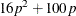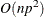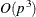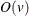### Computational Resources

Let p be the number of parameters estimated in the model. The minimum working space (in bytes) needed isHowever, if sufficient space is available, the input data set is also kept in memory; otherwise, the input data set is reread for each evaluation of the likelihood function and its derivatives, with the resulting execution time of the procedure substantially increased.

Let n be the number of observations used in the model estimation. Each evaluation of the likelihood function and its first and second derivatives requiresmultiplications and additions, n individual function evaluations for the log density or log distribution function, and n evaluations of the first and second derivatives of the function. The calculation of each updating step from the gradient and Hessian requiresmultiplications and additions. Thenotation means that, for large values of the argument, v,is approximately a constant times v.